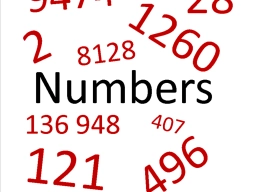# The sum 38

The sum of two decimal numbers is four. Their difference is one. What are the numbers?

a =  2.5
b =  1.5

### Step-by-step explanation:Did you find an error or inaccuracy? Feel free to write us. Thank you!

Tips for related online calculators
Do you have a linear equation or system of equations and looking for its solution? Or do you have a quadratic equation?